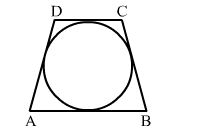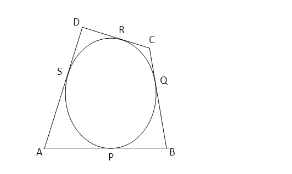# In the given figure, a circle touches all the four sides of a quadrilateral ABCD whose three sides are AB = 6 cm,

Question:

In the given figure, a circle touches all the four sides of a quadrilateral ABCD whose three sides are AB = 6 cm, BC = 7 cm and CD = 4 cm. Find AD.Solution:Let the circle touch the sides of the quadrilateral $A B, B C, C D$ and $D A$ at $P, Q, R$ and $S$ respectively.

Given, $A B=6 \mathrm{~cm}, B C=7 \mathrm{~cm}$ and $C D=4 \mathrm{~cm}$.

Tangents drawn from an external point are equal.

$\therefore A P=A S, B P=B Q, C R=C Q$ and $D R=D S$

Now, $A B+C D=(A P+B P)+(C R+D R)$

$=>A B+C D=(A S+B Q)+(C Q+D S)$

$=>A B+C D=(A S+D S)+(B Q+C Q)$

$=>A B+C D=A D+B C$

$=>A D=(A B+C D)-B C$

$=>A D=(6+4)-7$

$=>A D=3 \mathrm{~cm} .$

$\therefore$ The length of $A D$ is $3 \mathrm{~cm}$.